Next: The Continuum Hypothesis Up: Axiom of Choice and Previous: Relevance of the Axiom

# Cutting a sphere into pieces of larger volume

Is it possible to cut a sphere into a finite number of pieces and reassemble into a solid of twice the volume?

This question has many variants and it is best answered explicitly.

Given two polygons of the same area, is it always possible to dissect one into a finite number of pieces which can be reassembled into a replica of the other?

Dissection theory is extensive. In such questions one needs to specify

• What is a ``piece"? (polygon? Topological disk? Borel-set? Lebesgue-measurable set? Arbitrary?)
• How many pieces are permitted (finitely many? countably? uncountably?)
• What motions are allowed in ``reassembling" (translations? rotations? orientation-reversing maps? isometries? affine maps? homotheties? arbitrary continuous images? etc.)
• How the pieces are permitted to be glued together. The simplest notion is that they must be disjoint. If the pieces are polygons [or any piece with a nice boundary] you can permit them to be glued along their boundaries, ie the interiors of the pieces disjoint, and their union is the desired figure.

Some dissection results

• We are permitted to cut into finitely many polygons, to translate and rotate the pieces, and to glue along boundaries; then yes, any two equal-area polygons are equi-decomposable.

This theorem was proven by Bolyai and Gerwien independently, and has undoubtedly been independently rediscovered many times. I would not be surprised if the Greeks knew this.

The Hadwiger-Glur theorem implies that any two equal-area polygons are equi-decomposable using only translations and rotations by 180 degrees.

•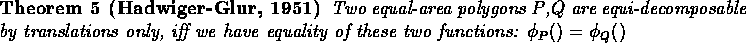Here, for each direction v (ie, each vector on the unit circle in the plane), let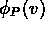be the sum of the lengths of the edges of P which are perpendicular to v, where for such an edge, its length is positive if v is an outward normal to the edge and is negative if v is an inward normal to the edge.

• In dimension 3, the famous ``Hilbert's third problem" is:
If P and Q are two polyhedra of equal volume, are they equi-decomposable by means of translations and rotations, by cutting into finitely many sub-polyhedra, and gluing along boundaries?

The answer is no and was proven by Dehn in 1900, just a few months after the problem was posed. (Ueber raumgleiche polyeder, Goettinger Nachrichten 1900, 345-354). It was the first of Hilbert's problems to be solved. The proof is nontrivial but does not use the axiom of choice.

References

Hilbert's Third Problem. V.G. Boltianskii. Wiley 1978.

• Using the axiom of choice on non-countable sets, you can prove that a solid sphere can be dissected into a finite number of pieces that can be reassembled to two solid spheres, each of same volume of the original. No more than nine pieces are needed.

The minimum possible number of pieces is five. (It's quite easy to show that four will not suffice). There is a particular dissection in which one of the five pieces is the single center point of the original sphere, and the other four pieces A,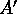, B,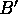are such that A is congruent toand B is congruent to. [See Wagon's book].

This construction is known as the Banach-Tarski paradox or the Banach-Tarski-Hausdorff paradox (Hausdorff did an early version of it). The ``pieces" here are non-measurable sets, and they are assembled disjointly (they are not glued together along a boundary, unlike the situation in Bolyai's thm.) An excellent book on Banach-Tarski is:

The Banach-Tarski Paradox. Stan Wagon. Cambridge University Press, 985

Robert M. French. The Banach-Tarski theorem. The Mathematical Intelligencer, 10 (1988) 21-28.

The pieces are not (Lebesgue) measurable, since measure is preserved by rigid motion. Since the pieces are non-measurable, they do not have reasonable boundaries. For example, it is likely that each piece's topological-boundary is the entire ball.

The full Banach-Tarski paradox is stronger than just doubling the ball. It states:

• Any two bounded subsets (of 3-space) with non-empty interior, are equi-decomposable by translations and rotations.

This is usually illustrated by observing that a pea can be cut up into finitely pieces and reassembled into the Earth.

The easiest decomposition ``paradox" was observed first by Hausdorff:

• The unit interval can be cut up into countably many pieces which, by translation only, can be reassembled into the interval of length 2.

This result is, nowadays, trivial, and is the standard example of a non-measurable set, taught in a beginning graduate class on measure theory.

•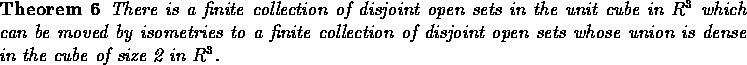This result is by Foreman and Dougherty.

• A square cannot be rearranged into a disk, if one is allowed finitely many pieces with analytic boundaries, glued at edges.
• A square can be rearranged into a disk, with translations only, if one is allowed to use finitely many pieces with unconstrained shape (not necessarily connected), and disjoint assembly.

References

Boltyanskii. Equivalent and equidecomposable figures. in Topics in Mathematics published by D.C. HEATH AND CO., Boston.

Dubins, Hirsch and ? Scissor Congruence American Mathematical Monthly.

``Banach and Tarski had hoped that the physical absurdity of this theorem would encourage mathematicians to discard AC. They were dismayed when the response of the math community was `Isn't AC great? How else could we get such counterintuitive results?' ''Next: The Continuum Hypothesis Up: Axiom of Choice and Previous: Relevance of the Axiom

Alex Lopez-Ortiz
Mon Feb 23 16:26:48 EST 1998